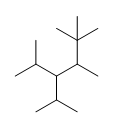# 3.1: Degree of Unsaturation: Another Aid to Identifying Molecular Structure

Objectives

After completing this section, you should be able to

1. determine the degree of unsaturation of an organic compound, given its molecular formula, and hence determine the number of double bonds, triple bonds and rings present in the compound.
2. draw all the possible isomers that correspond to a given molecular formula containing only carbon (up to a maximum of six atoms) and hydrogen.
3. draw a specified number of isomers that correspond to a given molecular formula containing carbon, hydrogen, and possibly other elements, such as oxygen, nitrogen and the halogens.

Key Terms

Make certain that you can define, and use in context, the key terms below.

• degree of unsaturation
• saturated
• unsaturated

There are many ways one can go about determining the structure of an unknown organic molecule. Although, nuclear magnetic resonance (NMR) and infrared radiation (IR) are the primary ways of determining molecular structures, calculating the degrees of unsaturation is useful information since knowing the degrees of unsaturation make it easier for one to figure out the molecular structure; it helps one double-check the number of $$\pi$$ bonds and/or cyclic rings.

## Saturated and Unsaturated Molecules

In the lab, saturation may be thought of as the point when a solution cannot dissolve anymore of a substance added to it. In terms of degrees of unsaturation, a molecule only containing single bonds with no rings is considered saturated.

 CH3CH2CH31-methyoxypentane

Unlike saturated molecules, unsaturated molecules contain double bond(s), triple bond(s) and/or ring(s).

 CH3CH=CHCH33-chloro-5-octyne

## Calculating The Degree of Unsaturation (DoU)

If the molecular formula is given, plug in the numbers into this formula:

$DoU= \dfrac{2C+2+N-X-H}{2} \tag{7.2.1}$

• $$C$$ is the number of carbons
• $$N$$ is the number of nitrogens
• $$X$$ is the number of halogens (F, Cl, Br, I)
• $$H$$ is the number of hydrogens

As stated before, a saturated molecule contains only single bonds and no rings. Another way of interpreting this is that a saturated molecule has the maximum number of hydrogen atoms possible to be an acyclic alkane. Thus, the number of hydrogens can be represented by 2C+2, which is the general molecular representation of an alkane. As an example, for the molecular formula C3H4 the number of actual hydrogens needed for the compound to be saturated is 8 [2C+2=(2x3)+2=8]. The compound needs 4 more hydrogens in order to be fully saturated (expected number of hydrogens-observed number of hydrogens=8-4=4). Degrees of unsaturation is equal to 2, or half the number of hydrogens the molecule needs to be classified as saturated. Hence, the DoB formula divides by 2. The formula subtracts the number of X's because a halogen (X) replaces a hydrogen in a compound. For instance, in chloroethane, C2H5Cl, there is one less hydrogen compared to ethane, C2H6.

For a compound to be saturated, there is one more hydrogen in a molecule when nitrogen is present. Therefore, we add the number of nitrogens (N). This can be seen with C3H9N compared to C3H8. Oxygen and sulfur are not included in the formula because saturation is unaffected by these elements. As seen in alcohols, the same number of hydrogens in ethanol, C2H5OH, matches the number of hydrogens in ethane, C2H6.

The following chart illustrates the possible combinations of the number of double bond(s), triple bond(s), and/or ring(s) for a given degree of unsaturation. Each row corresponds to a different combination.

• One degree of unsaturation is equivalent to 1 ring or 1 double bond (1 $$\pi$$ bond).
• Two degrees of unsaturation is equivalent to 2 double bonds, 1 ring and 1 double bond, 2 rings, or 1 triple bond (2 $$\pi$$ bonds).
DoU
Possible combinations of rings/ bonds

# of rings
# of double bonds
# of triple bonds
1
1
0
0

0
1
0
2
2
0
0

0
2
0

0
0
1

1
1
0

Remember, the degrees of unsaturation only gives the sum of double bonds, triple bonds and/or rings. For instance, a degree of unsaturation of 3 can contain 3 rings, 2 rings+1 double bond, 1 ring+2 double bonds, 1 ring+1 triple bond, 1 double bond+1 triple bond, or 3 double bonds.

Example 7.2.1: Benzene

What is the Degree of Unsaturation for Benzene?

Solution

The molecular formula for benzene is C6H6. Thus,

DoU= 4, where C=6, N=0,X=0, and H=6. 1 DoB can equal 1 ring or 1 double bond. This corresponds to benzene containing 1 ring and 3 double bonds.However, when given the molecular formula C6H6, benzene is only one of many possible structures (isomers). The following structures all have DoB of 4 and have the same molecular formula as benzene.## References

1. Vollhardt, K. P.C. & Shore, N. (2007). Organic Chemistry (5thEd.). New York: W. H. Freeman. (473-474)
2. Shore, N. (2007). Study Guide and Solutions Manual for Organic Chemistry (5th Ed.). New York: W.H. Freeman. (201)

Exercise $$\PageIndex{1}$$

Are the following molecules saturated or unsaturated:

(a.)(b.)(c.)(d.) C10H6N4